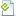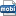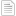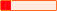#### Torrent DetailsNAME
Hands-On Mathematics for Deep Learning Build a solid mathematical foundation for training efficient deep neural networks.torrent
CATEGORY
Other
INFOHASH
d1df0ff27657bfdc30d46b9ff07c1f4e769b6814
SIZE
316 MB in 4 files
Uploaded on 28-06-2020 by our crawler pet called "Spidey".
SWARM
0 seeders & 0 peers
RATING

#### Description

Visit Site: FreePaidBooks.online

Hands-On Mathematics for Deep Learning: Build a solid mathematical foundation for training efficient deep neural networks

English | 2020 | 364 Pages | True PDF, EPUB, MOBI | 271.29 MB

A comprehensive guide to getting well-versed with the mathematical techniques for building modern deep learning architectures
Key Features

Understand linear algebra, calculus, gradient algorithms, and other concepts essential for training deep neural networks
Learn the mathematical concepts needed to understand how deep learning models function
Use deep learning for solving problems related to vision, image, text, and sequence applications

Book Description

Most programmers and data scientists struggle with mathematics, having either overlooked or forgotten core mathematical concepts. This book uses Python libraries to help you understand the math required to build deep learning (DL) models.

You’ll begin by learning about core mathematical and modern computational techniques used to design and implement DL algorithms. This book will cover essential topics, such as linear algebra, eigenvalues and eigenvectors, the singular value decomposition concept, and gradient algorithms, to help you understand how to train deep neural networks. Later chapters focus on important neural networks, such as the linear neural network and multilayer perceptrons, with a primary focus on helping you learn how each model works. As you advance, you will delve into the math used for regularization, multi-layered DL, forward propagation, optimization, and backpropagation techniques to understand what it takes to build full-fledged DL models. Finally, you’ll explore CNN, recurrent neural network (RNN), and GAN models and their application.

By the end of this book, you’ll have built a strong foundation in neural networks and DL mathematical concepts, which will help you to confidently research and build custom models in DL.
What you will learn

Understand the key mathematical concepts for building neural network models
Discover core multivariable calculus concepts
Improve the performance of deep learning models using optimization techniques
Understand computational graphs and their importance in DL
Explore the backpropagation algorithm to reduce output error
Cover DL algorithms such as convolutional neural networks (CNNs), sequence models, and generative adversarial networks (GANs)

Who this book is for

This book is for data scientists, machine learning developers, aspiring deep learning developers, or anyone who wants to understand the foundation of deep learning by learning the math behind it. Working knowledge of the Python programming language and machine learning basics is required.

Linear Algebra
Vector Calculus
Probability and Statistics
Optimization
Graph Theory
Linear Neural Networks
Feedforward Neural Networks
Regularization
Convolutional Neural Networks
Recurrent Neural Networks
Attention Mechanisms
Generative Models
Transfer and Meta Learning
Geometric Deep Learning

#### Files in this torrent

FILENAMESIZE9781838647292-HANDSON_MATHEMATICS_FOR_DEEP_LEARNING.pdf50.7 MB9781838647292.epub83 MB9781838647292.mobi181.9 MB[FreePaidBooks.online] Join for free ebooks!.txt126 B

#### Alternative Torrents for 'Hands Mathematics for Deep Learning Build solid mathematical foundation for training efficient deep neural networks'.7/1/2020286 MB006/28/2020316 MB00